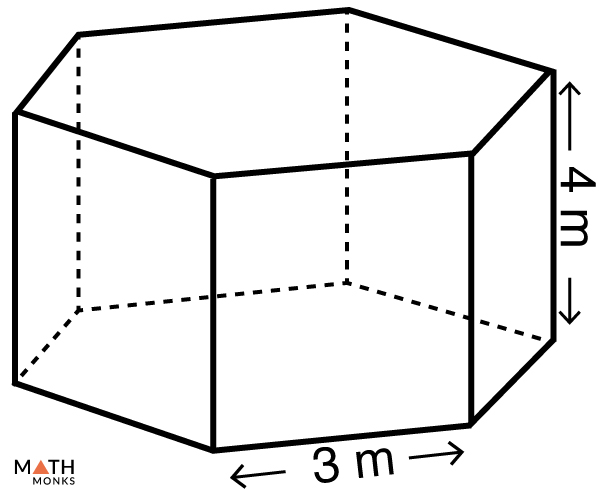# Surface Area of a Prism

The surface area, also known as the total surface area (TSA) of a prism, is the total space occupied by its flat faces. The surface area is measured in square units such as m2, cm2, mm2, or in2.

## Formulas

The general formula to find the total surface area of a prism is:

Total Surface Area (TSA) = 2 × Base Area + Base Perimeter × Height, here, the height of a prism is the distance between the two bases.

The total surface area of a prism is the combined area of the 2 bases and the areas of the lateral faces.

Since Lateral Surface Area (LSA) of a prism = Base perimeter x height

The lateral surface area is the area of all the faces except the bases.

We can also write:

Total Surface Area (TSA) = 2 × Base Area + LSA

However, there are specific formulas to calculate the surface area of different prisms. They are given below:

Some formulas have additional labeling for particular prisms.

In triangular, rectangular, and trapezoidal prisms, ‘l’ (or length) stands for the distance between the bases, and ‘h’ stands for the height of the polygonal base.  ‘l’ is the length for a square prism, and ‘a’ represents the four congruent base edges. For pentagonal and hexagonal prisms, ‘a‘ is the apothem, and ‘b’ is the base edge.

Let us solve some examples involving the above formulas to understand the concept better.

## Solved Examples

Find the total surface area of a triangular prism whose base edges are 4 cm, 4 cm, 3 cm, height is 3.71 cm, and length is 6 cm.

Solution:

As we know,
Total Surface Area (TSA) = b × h + (c) × l, here a = c = 4 cm, b = 3 cm, h = 3.71 cm, l = 6 cm
∴ TSA = 3 × 3.71 + (4 + 3 + 4) × 6
= 77.13 cm2Find the total surface area of a hexagonal prism whose base edge is 3 cm, apothem is 2.6 cm and height is 4 cm.Solution:

As we know,
Total Surface Area (TSA) = 6ab + 6bh, here = 6.5 cm, b = 3 cm, = 4 cm
∴ TSA = 6 × 2.6 × 3 + 6 × 3 × 4
= 6 × 3(2.6 + 4)
= 118.8 cm2

Find the total surface area of a rectangular prism with a length of 7 cm, a width of 4 cm, and a height of 5 cm.

Solution:

As we know,
Total Surface Area (TSA) = 2(lw + wh + lh), here l = 7cm, = 4cm, = 5 cm
∴ TSA = 2(7 × 4 + 4 × 5 + 7 × 5)
= 166 cm2

• More Resources: